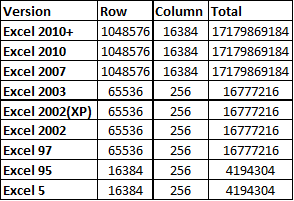# How many sheet,cells,rows and columns in one Excel

Jan 11, 2020 • edited Jan 12, 2020it depends on the Excel version you are using.

The number of cells, columns, rows, and sheets supported by a spreadsheet depend on the software being used, and the data contained in the spreadsheet. Below is a list of the maximum number of rows, columns, cells, and individual sheets supported by various spreadsheet software.

VersionRowsColumnsCellsSheets
Excel 20191,048,57616,384 XFD17,179,869,184*
Excel 20161,048,57616,384 XFD17,179,869,184*
Excel 20131,048,57616,384 XFD17,179,869,184*
Excel 20071,048,57616,384 XFD17,179,869,184*
Excel 2003 and earlier65,53625616,777,216*
OpenOffice Calc 31,048,57616,38417,179,869,184256
OpenOffice Calc 265,53625616,777,216256
Gnumeric65,53625616,777,216*
Lotus 1-2-365,53625616,777,216256
LibreOffice Calc1,048,5761,0241,073,741,82410,000

For MS Excel 2010, Row numbers ranges from 1 to 1048576; in total 1048576 rows, and Columns ranges from A to XFD; in total 16384 columns.

There are 220=1,048,576

rows, and 214=16,384

columns in an an Excel worksheet, at least since Excel 2007.

To find out how many rows, you can open an empty worksheet, and press CTRL-Down-Arrow key combination on your keyboard. The cursor will move all the way to the bottom row of the sheet. Look for the row number on the left.

To find out how many columns, you can press CTRL-Right-Arrow key combination in an empty worksheet, and you will find yourself at column XFD. So how many columns is that?

There are 26

columns labeled A through Z, and then there are 262=676 columns labeled AA through AZ. Then start the columns with 3-letter names. Treat AAA like 000 in base 26 arithmetic. Treat A as the 0 digit, and Z as the 25 digit in base 26 numerals. So X is the 23 digit, F is the 5 digit, and D is the 3 digit, and XFD is the base-26 for 23×262+5×26+3×1=15,681

. So the three-letter column names run from 0 to 15,681, so there are 15,682 of them.

So altogether there are 26 + 676 + 15,682 = 16,384 columns.

#Tips#Rows #Columns

250+ Excel Keyboards Shortcuts List for you

How to Count Blank/Nonblank Cells